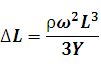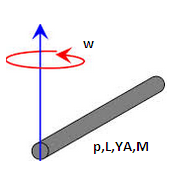# Elongation in a rotating rod Calculator

This Calctown Calculator calculates the elongation due to strain in a rotating rod fixed at one end.

kg/m3
m
rad/s
Pa

#### Result

mClick here to view image

where

ΔL = elongation in rod

L =  lenght of rod

ρ = density of rod

ω = angular velocity of rod

Y = young's modulus of rod